# Percolation

Written by Paul Bourke
April 2022

The following structures have been used to study porosity and percolation as they offer a controlled means of creating 3D structures of known fractal dimension that can be used in fluid flow simulations. While the details may vary between implementations, the underlying algorithm is particularly simple. It can be implemented in any dimension, here the 3D case is considered. One starts with a cuboid which on the first iteration is split M times in each dimension, this results in M3 smaller cuboids. Each of these cuboids are either retained or discarded depending on some constant probability P, typically from a uniform distribution on [0,1]. In subsequent iterations the process is repeated for each remaining cuboid. In this the simplest version, there are only four parameters (M,P,N,S), where S is the random number seed for the probabilities and N is the number of iterations performed which determines the resolution/detail of the resulting structure. The statistical nature of the structure is determined by just two parameters, (M,P).

An example of a sequence from N=1 to N=8 (N=0 is just the unit cube), M=2, P=0.62 is shown below.

The following images show the 3D structures for various other combinations of M and P. Obviously, P controls the density and connectedness of the resulting structure, with the phase change between a disjoint set and connected set occuring around P = 0.65.P = 0.55P = 0.6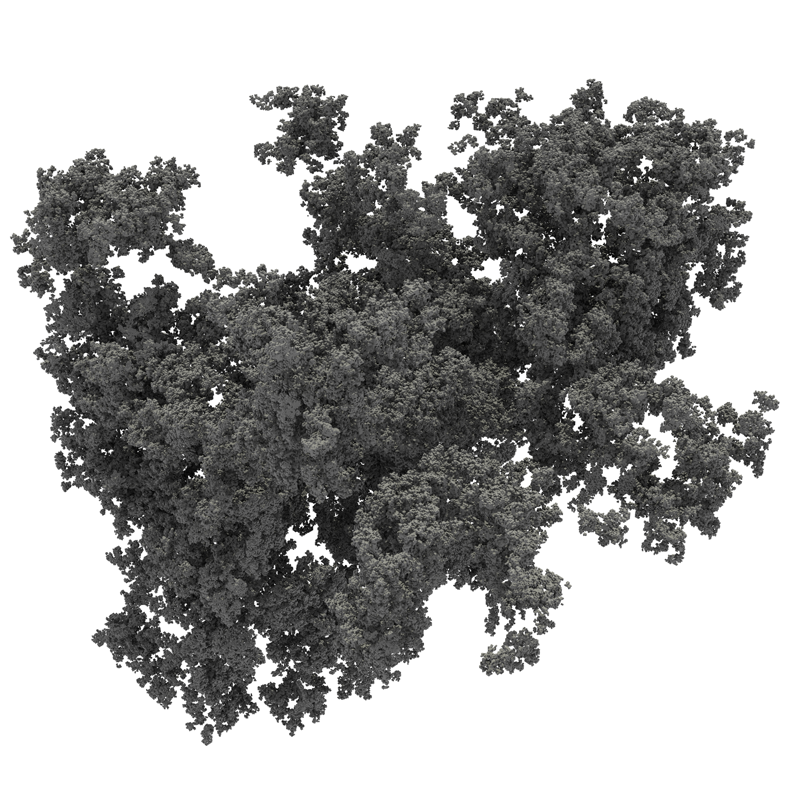P = 0.6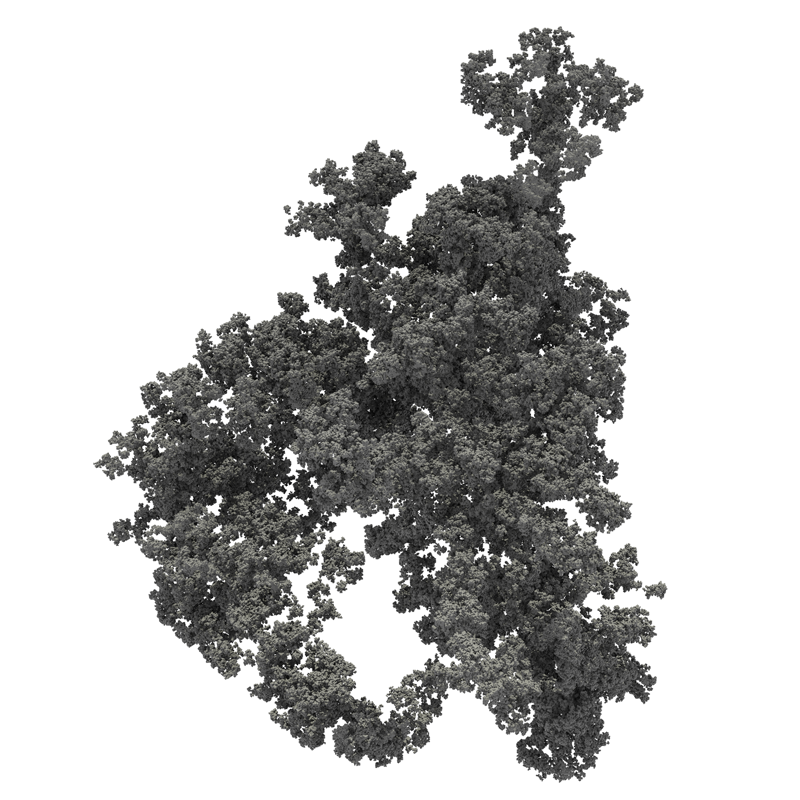P = 0.62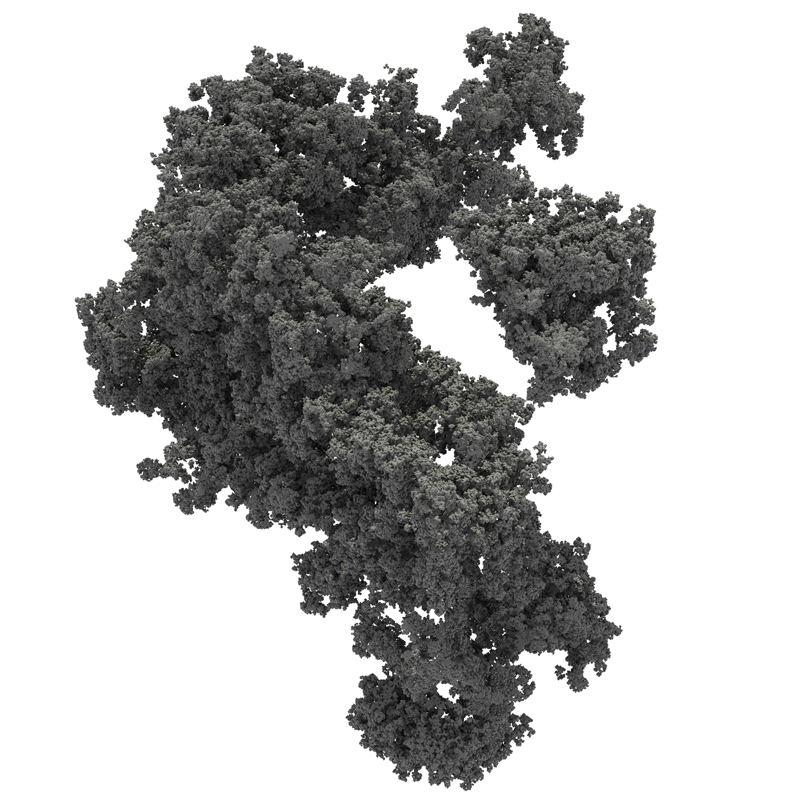P = 0.65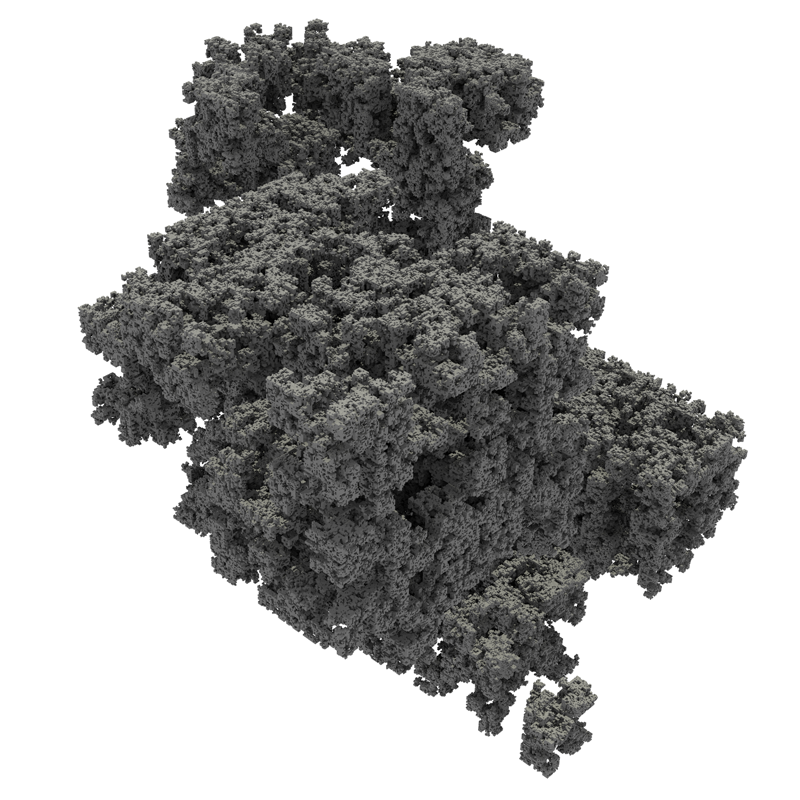P = 0.75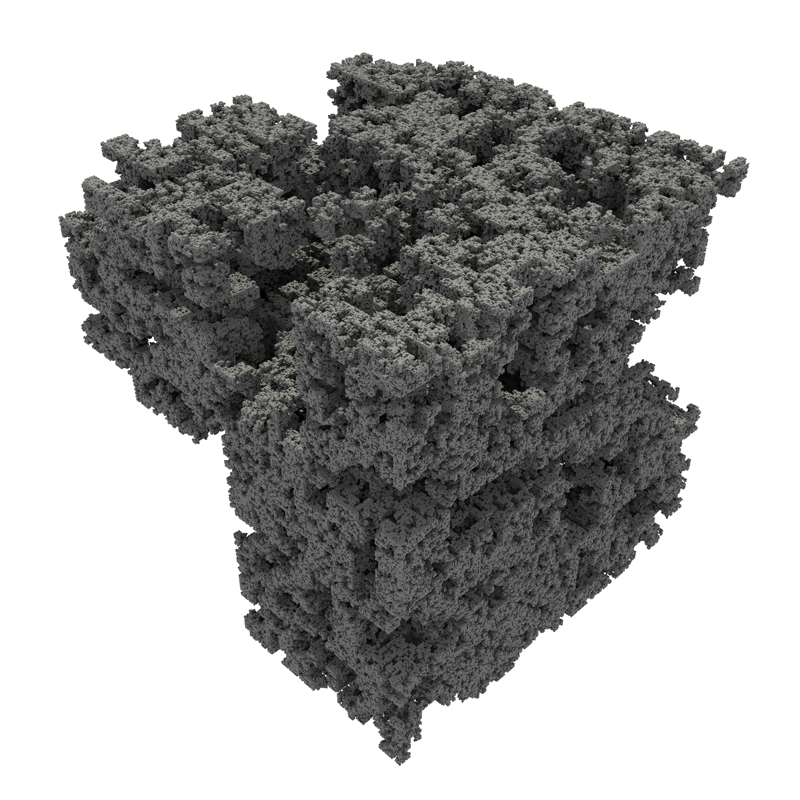P = 0.8